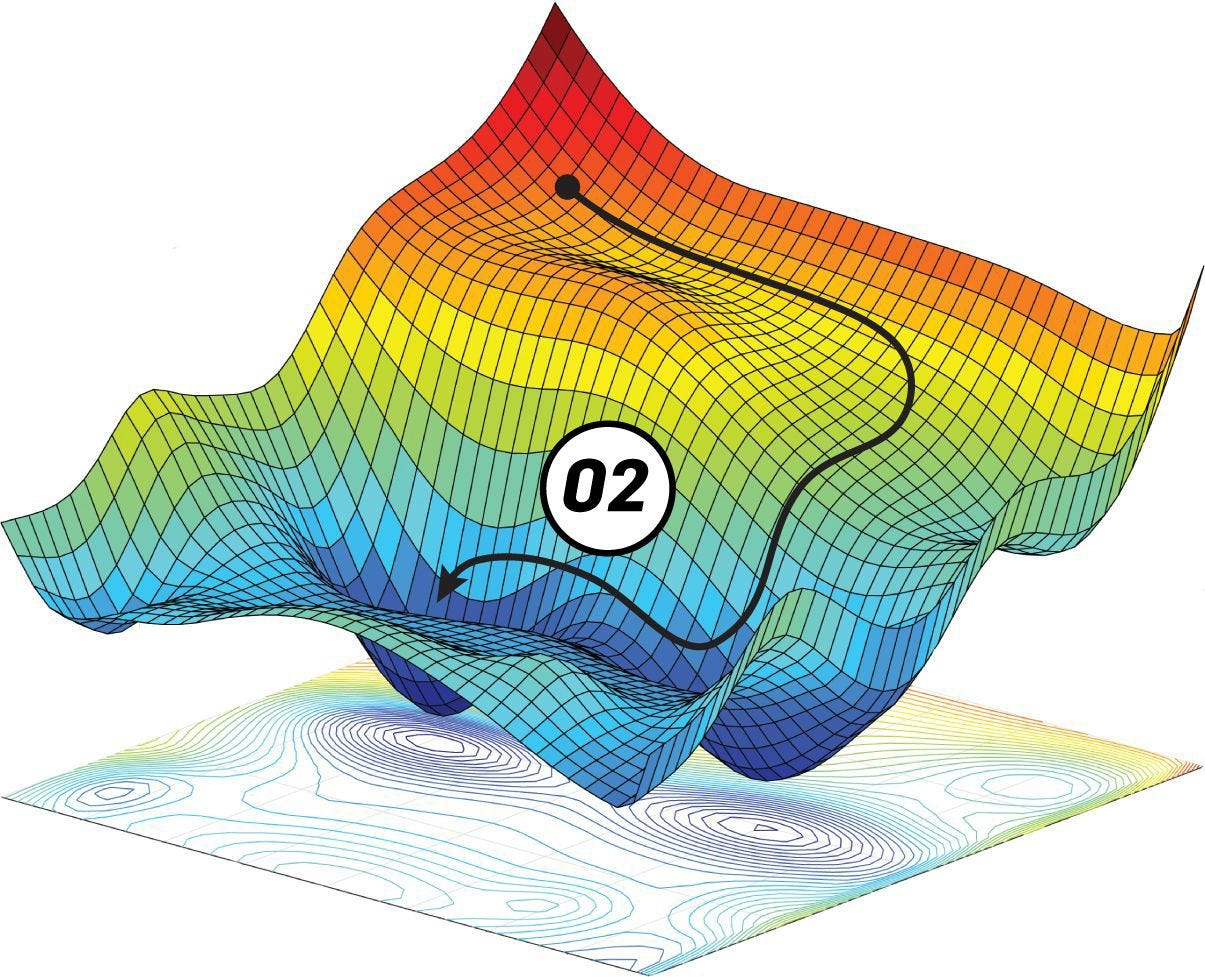Gradient descent is a generic optimization algorithm that tweaks parameters iteratively to minimize a cost function.

Suppose you are lost in the mountains when it is pitch dark , and you can only feel your movement on the ground below. Now, If you are looking to get to the bottom of the valley or the mountain ,the best strategy is to move towards the steepest slope . This is exactly what the gradient descent does, it measures the local gradient of the error function with regard to the parameter vector(θ) ,and it goes in the direction of the descending gradient . We hit the minimum once the gradient is zero.

We start by initializing weight (θ) with random values ,this is also called as random initialization.Then we move towards the minima , i.e we take baby steps in an attempt to decrease the cost function , until the algorithm converges to a minimum value.This is illustrated in the image above.

A very important parameter in gradient descent is the size of the steps taken. This is determined by the learning rate hyper parameter. Having either very low learning rate or very high learning rate is not advisable. Let us see why.

If the learning rate is too low, the algorithm will go through many iterations to converge ,which will take a long time.

Whereas , if the learning rate is too high , the algorithm might diverge,you may end up on the other side of the curve ,possibly higher as well .The algorithm may never converge to the minima that’s expected.

Not all cost functions are convex in nature, it may have multiple ridges. i.e they can have multiple local minima and a global minima.This is illustrated in the image above.

If the random initialization starts from the left ,the algorithm might converge to a local minima , we may never reach the global minimum.

On the other hand , if we start from the right , the algorithm might take a long time to cross the plateau,in the worst case scenario ,the algorithm may stop early , and may never reach the global minimum.

Training a model means searching for a combination of model parameters that minimizes a cost function.Which means , searching for global minimum of the cost function.In some cases , like linear regression , it is easier as the cost function is usually convex function ,in which case the function has just one global minima and no local minima.

Gradient descent is given by -

Where η is known as the learning rate, which defines the speed at which we want to move towards negative of the gradient. Let’s try to understand this.